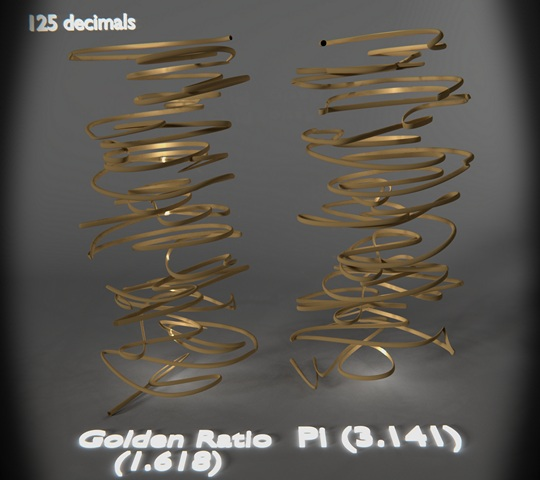# Pi as a sculpture

Pi as a 3D sculpture

I recently wrote a python program in python’s IDLE which calculates pi using chudnovsky’s formula. Using the output of this the program takes each decimals of pi and translates it into coordinates around the edge of a circle. This is then exported to an .obj file and within Blender smoothing and lighting is added.

This image (The full one : http://goo.gl/6XGLUq) shows pi to *5000 (not 10000) decimals bottom to top, the computation of this, and it being saved to a .obj file, took 24 seconds. Rendering, a bit longer at 2 hours 30 mins, at 1600 samples.

### Attachments

Interesting to say the least, to see mathematical expressions and all that translated into the 3D world!

Thank-you -
I just though I should say that this image is to 5000 numbers of pi not 10000 as I mistakenly put before.

This looks charmingI’m curious. By which algorithm / method did you translate the calculated numbers into x/y/z coordinates? Would be nice if you could elaborate a bit on that if time allowsCertainly

X coordinate = Radius * cos( 360 - (last x) + 36* (Pi Decimal))
Y coordinate = Radius * sin( 360 - (last y) + 36* (Pi Decimal))
z coordinate = Iteration (0,1,2…) * height + (Pi Decimal/100)

36 is used as there are 10 possible decimal numbers, 0 to 9,

Thinking about it I did’nt actually need the 360 - part becuase of laws of cos and sin. However, I used the last positions so that I can offset the new position slightly (stops most overlap) It does not massivly impact the output however.
The z is mostly about the same each new decimal with only slight variations.

Very cool.

125 digits of each; I show pi and the golden ratio beside each other:### AttachmentsAwesome, thanks for the explanation. A fascinating way to create geometry from numerical input!

Thank-you!

Awesome. Awesome. Awesome. Awesome. Awesome. Awesome. Awesome. Awesome. Awesome. Awesome. Awesome. Awesome. Awesome. Awesome. Awesome. Awesome. Awesome. Awesome. Awesome. Awesome. Awesome. Awesome. Awesome. Awesome. Awesome. Awesome. Awesome. Awesome. Awesome. Awesome. Awesome. Awesome. Awesome. Awesome. Awesome. Awesome. Awesome. Awesome. Awesome. Awesome. Awesome. Awesome. Awesome. Awesome. Awesome. Awesome. Awesome. Awesome. Awesome. Awesome. Awesome. Awesome. Awesome. Awesome. Awesome. Awesome. Awesome. Awesome. Awesome. Awesome. Awesome. Awesome. Awesome. Awesome. Really. As a piece of abstract art this is so cool. No complaints.

the thumbnail view makes me think it is the shadow of mirror in river

@@Blender2003
Thank you!
@@Oyster
I can see that, it is the colour, but pi is everywhere!

looks like gyros to me! well done!

You should up the level of detail and then 3D print it. They’d like it at one of them Modern Art Museums. I think!

I have always loved geometrical representations of of numbers like Pi. This is very interesting. Maybe is there a way to improve the esthetic ?

Do you think you could post your python code?

@JosephBburg It would be a challenge to do! I don’t have a printer for that though…
@Paponetman What ideas would you have?
@Nicholas_A The code is not written great… but yes, you can have it: The program should run in the IDLE, to get the obj file to import properly, you will need to check ‘Keep Vert Order’. Then You can convert it to a curve so you can have a solid shape.

``````
from decimal import *
import math
import sys
import time
from functools import lru_cache as cache

cache(maxsize=None)

sys.setrecursionlimit(25000)

numberOfPi = int(input("Number of Pie "))
getcontext().prec = numberOfPi

def factorial(n):
if n&lt;1:
return 1
else:
return n * factorial(n-1)

def chudnovskyBig(n): #http://en.wikipedia.org/wiki/Chudnovsky_algorithm http://blog.recursiveprocess.com/2013/03/14/calculate-pi-with-python/

pi = Decimal(0)
k = 0
while k &lt; n:
pi += (Decimal(-1)**k)*(Decimal(factorial(6*k))/((factorial(k)**3)*(factorial(3*k)))* (13591409+545140134*k)/(640320**(3*k)))
k += 1
if(k == numberOfPi/2):
print("Half Done")

pi = pi * Decimal(10005).sqrt()/4270934400
pi = pi**(-1)
#print(pi)
ToOBJ(pi)
return pi

def ToOBJ (p):
text_file = open("PiSculpture.obj", "w")
OBJ =''
ArPi = (list(str(p)))
ArPi.remove('.');
PrevV = (0,0,0)
SaveObj= 0
LsOBJ = [0,0]
ler = 0

for index in range(0,len(ArPi)):
w = 10
h = 0.01
# x = Radius * cos( 360 - (last x) + 36* (Pi Decimal))

OBJ = OBJ +'
v ' + str(LsOBJ) + ' ' + str(index*h + (int(ArPi[index])/100)) +' ' + str(LsOBJ)
ls = ''
print('Half done .obj')
for ler in range(0,len(ArPi)):
ler +=1

ls = ls + ('
l ' + str(ler) +' ' + str(ler+1))

text_file.write('o Pi 3D Shape
' + str(OBJ) +str(ls))
print('Saved')
text_file.close()

start = time.clock()
chudnovskyBig(int(0.069793*numberOfPi+0.732175)+6)
print ("Time take to do everything is : " + str(time.clock() - start))
``````